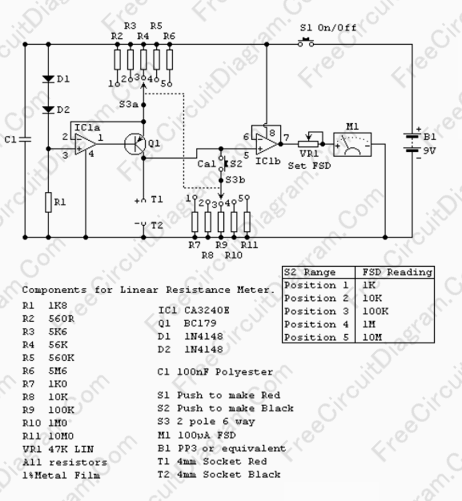# Using Current Source To Make Linear Scale Analog Ohm MeterMany analog ohm meters have non linear scale, so the resolution become worse at higher resistance value. This because they use cheap current source, only a series resistance to approximate an ideal current source. An ideal current source will force a consistent current amount regardless of the tested resistor value.  Using regulated current source, the current flowing through the tested resistor will be kept constant, so the measured voltage across the tested resistor is proportional (linear) to its resistance value. The circuit diagram is shown below:The current source is provided by IC1a. The two 1N4148 diodes give a constant reference, so the voltage across selected R2..R6 is constant at about 1.4 volt. The R1..R6 is selected to measure different resistor ranges. The VR1 has to be adjusted every time you switch for different range, just like as you do in your old classic analog ohmmeter.